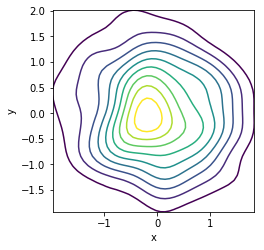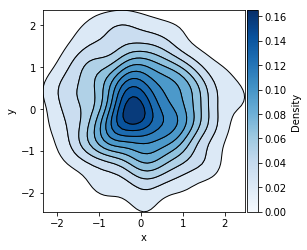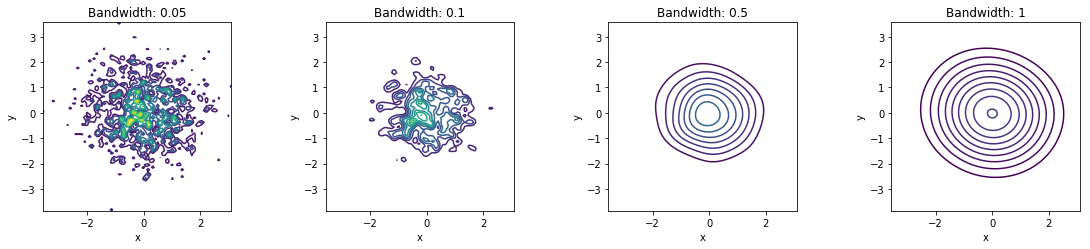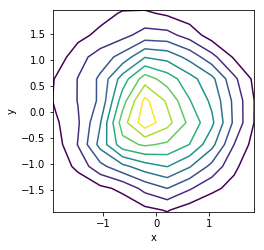Bivariate¶

Title
Bivariate Element
Dependencies
Matplotlib, SciPy
Backends
Matplotlib
Bokeh
In :
import numpy as np
import holoviews as hv

hv.extension('matplotlib')Bivariate provides a convenient way to visualize a 2D distribution of values as a Kernel density estimate and therefore provides a 2D extension to the Distribution element. Kernel density estimation is a non-parametric way to estimate the probability density function of a random variable.

The KDE works by placing a Gaussian kernel at each sample with the supplied bandwidth, which are then summed to produce the density estimate. By default the bandwidth is determined using the Scott's method, which usually produces good results, but it may be overridden by an explicit value.

To start with we will create a Bivariate with 1,000 normally distributed samples:

In :
normal = np.random.randn(1000, 2)
hv.Bivariate(normal)
Out:A Bivariate might be filled or not and we can define a cmap to control the coloring:

In :
hv.Bivariate(normal).opts(colorbar=True, cmap='Blues', filled=True)
Out:We can set explicit values for the bandwidth to see the effect. Since the densities will vary across the NdLayout we will enable axiswise normalization ensuring they are normalized separately:

In :
hv.NdLayout({bw: hv.Bivariate(normal).opts(axiswise=True, bandwidth=bw)
for bw in [0.05, 0.1, 0.5, 1]}, 'Bandwidth')
Out:Underlying the Bivariate element is the bivariate_kde operation, which computes the KDE for us automatically when we plot the element. We can also use this operation directly and print the output highlighting the fact that the operation simply returns an Contours or Polygons element. It also affords more control over the parameters letting us directly set not only the bandwidth and cut values but also a x_range, y_range, bw_method and the number of samples (n_samples) to approximate the KDE with:

In :
from holoviews.operation.stats import bivariate_kde
dist = hv.Bivariate(normal)
kde = bivariate_kde(dist, x_range=(-4, 4), y_range=(-4, 4), bw_method='silverman', n_samples=20)
kde
Out:For full documentation and the available style and plot options, use hv.help(hv.Bivariate).